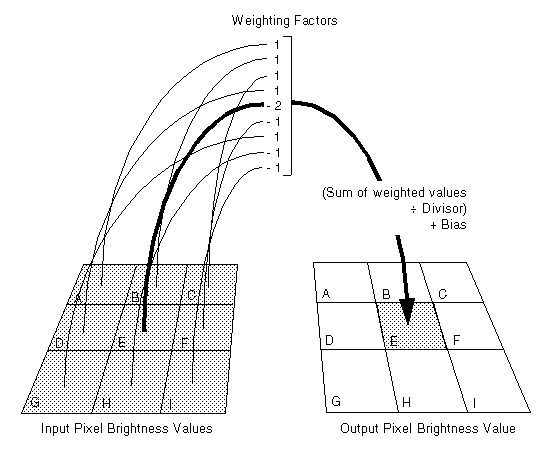﻿ Matrix Property (SpatialFilterCommand) | Leadtools.ImageProcessing.Effects | Raster, Medical, Document Help
←Select platform
In This Topic ▼

# Matrix Property

Summary

Gets or sets the array of weighting factors.

Syntax
C#
VB
Objective-C
C++
Java
``public int[] Matrix { get; set; } ``
``Public Property Matrix As Integer() ``
``@property (nonatomic, assign, nullable) const int *matrix ``
````public int[] getMatrix() `
`public void setMatrix(int[] value) ````
````public: `
`property array<int>^ Matrix { `
`   array<int>^ get(); `
`   void set (    array<int>^ ); `
`} ````

#### Property Value

The array of weighting factors. The factors apply to pixels in the neighborhood read left to right and top to bottom in the ABC order shown below:Example

Refer to SpatialFilterCommand()

Requirements